# Facenet: A Unified Embedding for Face Recognition and Clustering

19 May 2018

This is the eighth article for the website.

In this blog post, I will be writing a paper summary on FaceNet: A Unified Embedding for Face Recognition and Clustering by Florian Shroff, Dmitry Kalenichenko and James Philbin from Google. As in the previous blog articles, we will be employing the techniques highlighted in this as before.

1) What problem is the entire field trying to solve?

Ans. This field is trying to solve the triple problem of face recognition, verification and clustering. This paper is trying to find a universal solution to these problems.

2)Summary of the background

Ans. Face recognition has been a hot topic and substantial research has been done on it. Previous research employed the deep Conv2D networks which used a bottleneck Convolutional layer with a large number of hidden layers. This high dimensionality leads to inefficiency. Another drawback that we can observe is that the bottleneck convolutional layer does not generalize well with newer faces. To counter these limitations, the author have used a triplet loss based function on LMNNlarge margin nearest neighbour classification.

Digression: We will now take a digression to explain the important terms: LMNN and triplet loss based function.

1) LMNN: In the paper linked, the authors have noted the problems faced with KNN metric while measuring the distances. It neglects a lot of important statistical factors depending on the problem at hand. For example, for classifying disparate things like age and gender, we can’t use the same distance metric. The researchers have now used a more powerful technique which helps in calculating the distances of different class/ label, known as Mahalanobis metric

2) Triplet loss based function: In this methodology, we have three images, Anchor image(A), Positive image(P) and Negative image(N).Anchor imagePositive imageNegative image

The objective of our triplet loss based function is to minimize the L2 distance between the anchor image and the positive image and maximize the L2 distance between the anchor image and negative image.3)Identify specific questions.

Ans. In this approach, the authors are using the triplet loss based function to improve the task of face recognition over the previously employed methods and also solve the problems of verification and clustering. To succeed, we need to learn good triplets. The authors want to create a good method to make this possible.

4) Identify the approach.

Ans. To solve the previous problem of choosing appropriate triplets to improve learning, the researchers take a cue from human learning system. A human is subject to a curriculum which introduces concepts starting from easy to difficult slowly. For example, we learn addition in class 1 and slowly progress to multiplication and division in higher classes. This paradigm of learning, also known as curriculum leaning is used in choosing the appropriate triplets. We start from simpler images, and build upon them to finally learn more difficult triplet combinations.

5) Methods:

Ans. The authors have used existing network architectures like Zeiler and Feggus and Inception as black boxes in learning of the system.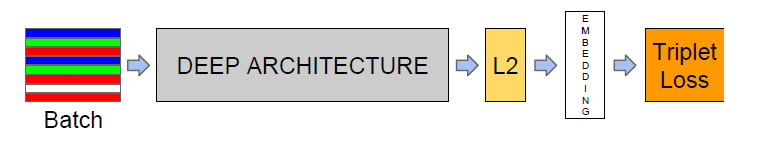Our primary objective is to minimize d(A,P) and maximize d(A,N), where d(X,Y) stands for the L2 squared distance between image X and image Y. For all the images, this can be summarized to minimize L: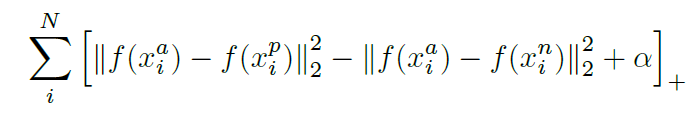Here, alpha is defined as a margin upto which we can tolerate d(A,P) and d(A,N).

Also, as we are using curriculum leaning, we try to divide our main goal( to minimize L) into sub goals( maximize d(A,P) and minimize d(A,N)). To prevent over-generalization of images, we train using mini-batches. To prevent another problem of achieving the local minima, we use this following heuristic: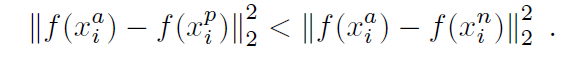6)Results:

Ans. The authors have summarized all the important results comparing various parameters like FLPS, size, no of embeddings and metrics like VAL(validation rate) and FAR(false accept rate )

All face pairs belonging to the same cluster( similar faces ) are denoted asand other remaining pairs are denoted as.

We define the true accepts as the set of pairs correctly classified as same at threshold d. Mathematically, we can define as: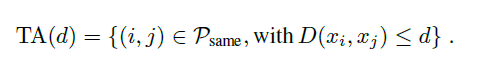Analogously, we can define false accepts as the set of pairs incorrectly classified as same. Mathematically, we can define as: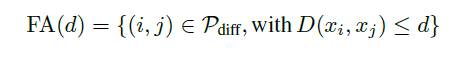Now, we can define VA(d) asand FAR(d) as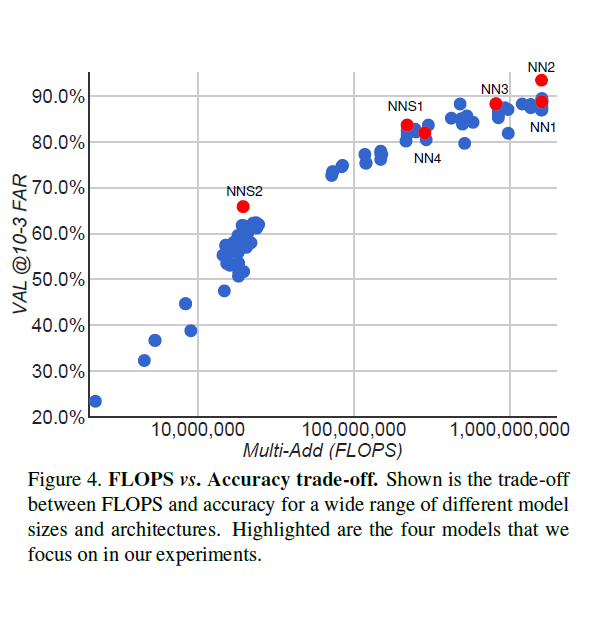In the above table, we compare the FLOPS vs accuracy tradeoff of different neural networks. We compare the Zeiler and Feggus network and the different modifications of the Inception network.

7) Determine whether the results answer the specific questions?

Ans. In this paper, the authors have given an improvement of face recognition over the existing methods. The authors tabulate their results and compare using many parameters like FLOPS, number of embeddings to find a suitable network for learning the triplets. This has helped the authors to remove the inflexibility present earlier. They have also proposed a better method to make the embeddings compatible over different datasets known as Harmonic embeddings. We use a combination of embeddings during the learning stage.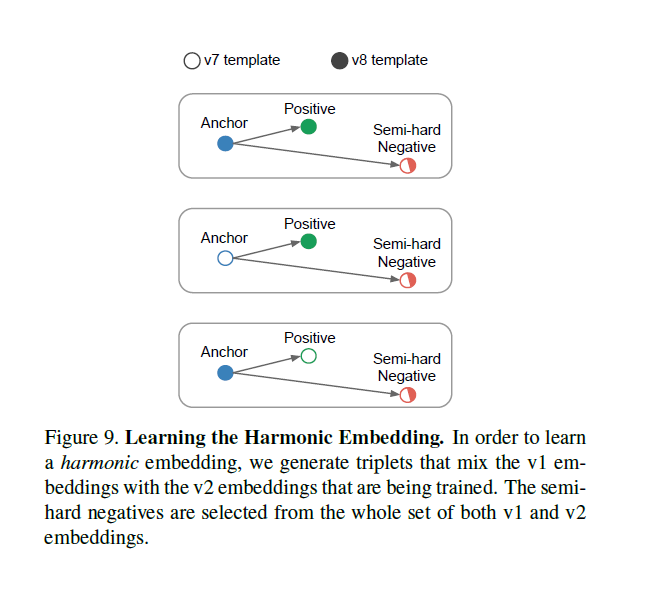In the following graph, we observe how using Harmonic embeddings changed the VAL vs FAR tradeoff.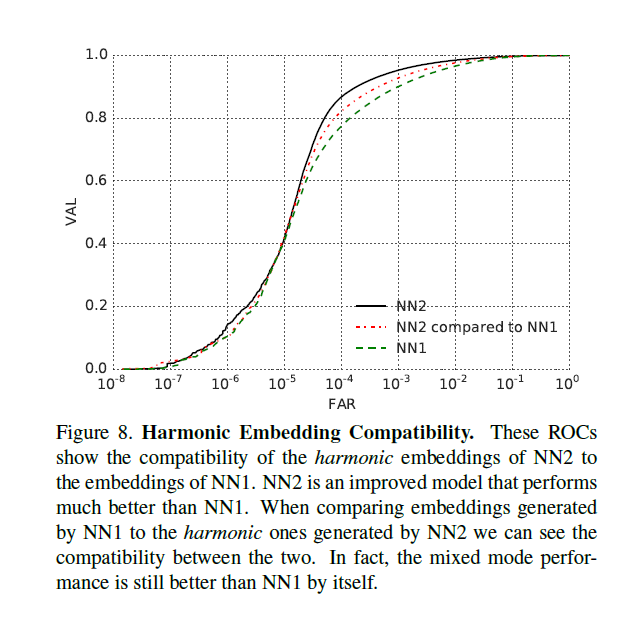I would love to hear feedback on the article. Any suggestions or mistakes can be pointed out in the comments below.